# Brass tube

The outer perimeter of brass tube (ρ = 8.5 g/cm3) is 38 cm. Its mass is 5 kg, length 54 cm. What is the pipe wall thickness?

h =  2.938 mm

### Step-by-step explanation: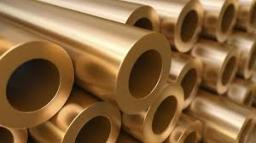Did you find an error or inaccuracy? Feel free to write us. Thank you!Tips to related online calculators
Do you want to convert length units?
Tip: Our Density units converter will help you with the conversion of density units.

## Related math problems and questions:

• Hollow sphereThe steel hollow sphere floats on the water plunged into half its volume. Determine the outer radius of the sphere and wall thickness, if you know that the weight of the sphere is 0.5 kg and the density of steel is 7850 kg/m3
• Steel tubeThe steel tube has an inner diameter of 4 cm and an outer diameter of 4.8 cm. The density of the steel is 7800 kg/m3. Calculate its length if it weighs 15 kg.
• Brass sphereFind the weight of a brass ball with an outer radius of 12 cm, a wall thickness of 20 mm if the brass's density is 8.5 g/cm3.
• Wall thicknessThe hollow metal ball has an outside diameter of 40 cm. Determine the wall thickness if the weight is 25 kg and the metal density is 8.45 g/cm3.
• Mass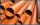The thickness of a metallic tube is 1cm, and its outer radius is 11cm. Find the mass of such a 1m long tube if the metal density is 7.5g per cubic cm.
• Plastic pipeCalculate the plastic pipe's weight with diameter d = 70 mm and length 380 cm if the wall thickness is 4 mm and the density of plastic is 1367 kg/m3.
• Iron densityCalculate the weight of a 2 m long rail pipe with an internal diameter of 10 cm and a wall thickness of 3 mm. The iron density is p = 7.8 g/cm3.
• The squareThe square oak board (with density ρ = 700 kg/m3) has a side length of 50 cm and a thickness of 30 mm. 4 holes with a diameter of 40 mm are drilled into the board. What is the weight of the board?
• Swing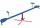A child weighing 12 kg is sitting on a swing at a distance of 130 cm from the rotation axis. How far away from the rotation (center) axis must sit down his mother weighs 57 kg if she wants to be a swing in balance?
• CuZn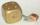Brass is an alloy of copper and zinc. The 10 centimeter brass cube has a weight of 8.6 kg. Copper density is 8930 kg/m3, the zinc density is 7130 kg/m3. Calculate how many kg of copper and zinc a cube contains.
• Brass ball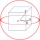What is the weight of a hollow brass ball, the outer diameter of which is 16 cm and the wall thickness is 2 cm, while the density of brass is ϱ = 8.5 g
• Brick wallWhat is the weight of a solid brick wall that is 30 cm wide, 4 m long and 2 m high? The density of the brick is 1500 kg per cubic meter.
• Copper wireWhat is the weight in kg of copper wire 200 m long with a diameter of 0.6 cm, if the density of copper is 8.8 kg?
• Cuboid 5Calculate the mass of the cuboid with dimensions of 12 cm; 0.8 dm and 100 mm made from spruce wood (density = 550 kg/m3).
• The pipe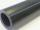The pipe is 1.5 m long. Its outer diameter is 60 cm, inner diameter is 52 cm. Calculate the pipe's weight if the material's density from which it is made is 2 g/cm3. Round the results to whole kilograms.
• A stone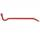There is a stone weighing 60 kg on the billet. The distance from the support point to the stone is 20cm. The length of the billet is 1m. Determine the force exerted by the hand at the end of the billet.
• SphereThe surface of the sphere is 12100 cm2, and the weight is 136 kg. What is its density?